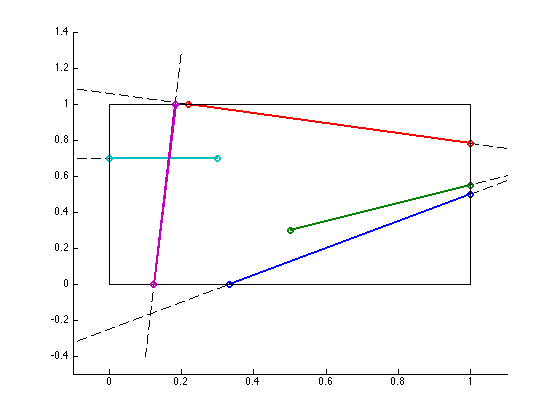This is so easy, someone else should have done it already. There is some code here: http://www.mathworks.co.uk/matlabcentral/fileexchange/25528-line-clipping. However, among other things (like using `input`), it doesn't check for lines parallel with the clipping window borders.

Wikipedia gives a variety of algorithms -- Liang/Barsky's algorithm is probably the one which one implement most quickly.

Here goes the code:

``function [xx1, xx2] = clipped_line(vx1, vx2, v1, v2)% function [xx1, xx2] = clipped_line(vx1, vx2, v1, v2)% % Liang-Barsky Algorithm for line clipping. See% http://en.wikipedia.org/wiki/Liang%E2%80%93Barsky_algorithm%% Take a 2-D line from vx1 to vx2, and clip to rectangle with corners at% v1, v2.% % Inputs must be vectors of length 2.% Outputs are vectors of length 2. If no outputs are specified, a plot is% made.%    if length(vx1) ~= 2 || length(vx2) ~= 2 || length(v1) ~= 2 || length(v2) ~= 2        error('All inputs must be vectors of length 2.');    end    x0 = vx1(1);    x1 = vx2(1);    y0 = vx1(2);    y1 = vx2(2);        x_min = min(v1(1), v2(1));    x_max = max(v1(1), v2(1));    y_min = min(v1(2), v2(2));    y_max = max(v1(2), v2(2));    xx1 = nan(size(vx1));    xx2 = nan(size(vx2));        dx = x1 - x0;    dy = y1 - y0;        p = [ -dx;            dx;           -dy;           dy ];    q = [ x0 - x_min;   % negative => left of window           x_max - x0;   % negative => right of window           y0 - y_min;   % negative => below window          y_max - y0;   % negative => above window        ];              % Test if line isn't visible.%     for i = 1:4%         if p(i) == 0 && q(i) < 0%             return;%         end%     end    if ~isempty(find(p == 0 & q < 0, 1, 'first'))        return;    end        u1 = 0;    u2 = 1;        for i = 1:4        if p(i) < 0            u1 = max(u1, q(i)/p(i));        end        if p(i) > 0            u2 = min(u2, q(i)/p(i));        end    end        if u1 > u2  % line is outside        return;    end        xx1(1) = x0 + dx*u1;    xx1(2) = y0 + dy*u1;    xx2(1) = x0 + dx*u2;    xx2(2) = y0 + dy*u2;    if nargout == 0        rectangle('position', [x_min y_min (x_max-x_min) (y_max-y_min)]);                l1 = line([x0 x1], [y0 y1]);        set(l1, 'color', 'k', 'linestyle', '--');        hold all        l2 = plot([xx1(1) xx2(1)], [xx1(2) xx2(2)], 'o-');        set(l2, 'linestyle', '-', 'linewidth', 2);                xlim([ min([x0, x1, x_min])-.1, max([x0, x1, x_max])+.1 ]);        ylim([ min([y0, y1, y_min])-.1, max([y0, y1, y_max])+.1 ]);    end    end``

Now, running this:

``figure; clipped_line([-1, -1], [3, 2], [0 0], [1 1]); clipped_line([0.5, 0.3], [1.3, 0.7], [0 0], [1 1]);clipped_line([-0.5, 1.2], [1.3, 0.7], [0 0], [1 1]);clipped_line([-0.5, 0.7], [0.3, 0.7], [0 0], [1 1]);clipped_line([0.2, 1.3], [0.1, -0.4], [0 0], [1 1]);``

Makes this: